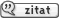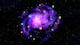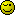Neue Frage » Antworten »
Autor Nachricht

Anmeldungsdatum: 26.03.2017
Beiträge: 1Verfasst am: 26. März 2017 14:54    Titel: ket in quantum physicsMeine Frage: Hi, I have a problem to understand what really is a ket, i know what is a hilbertspace, here we have skalarproduct and covergence of every caucy secuence. For a (normal) function with for example dependency of x,y,z, I understand this. But what is a ket, here we do not have any dependency from any stationary vector (as x,y,z). What should this be ? A matrix with entries ? What does it say ? and how can any operator be defined that can be applied on a ket ? for normal function f(x,y,z) this is clear. Every wave function is assigened to a ket how does this work, which information of wave function contains the ket vektor ? Hope someone can explain me Meine Ideen: I have suggestion, that ket is a matrix with entries of different values of the wave function on different places for stationary vector, because there are endless entries possible (stationary verctor is continious), the ket is a matrix (or row vector) with an endless numer of entries. But if this is case, we have a dependency from stationary vector in the ket matrix \left| n \right> =\begin{pmatrix}till -\infty \\f(x1) \\ f(x2) \\ f(x3) \\till +\infty \end{pmatrix}ModeratorAnmeldungsdatum: 10.07.2012
Beiträge: 8245Verfasst am: 26. März 2017 14:58    Titel:Have a look here: https://en.wikipedia.org/wiki/Braket_notation especially here: https://en.wikipedia.org/wiki/Braket_notation#Ket_notation_for_vectors and -for the connection to wave functions- here: https://en.wikipedia.org/wiki/Braket_notation#Spinless_position.E2.80.93space_wave_functionAnmeldungsdatum: 25.03.2017
Beiträge: 9Verfasst am: 26. März 2017 18:41    Titel: understood right ?When I understood it right, there is a hilbert space, in this space we have different Basis possilble, for exampleorwhere the first related to space, the second to impules. The ket can be expressed in both basises, it is the same object, but with different representatations in different basises. Because there are endless points in space, the dimension of hilbert space is infinite. The ket can be expressed in Basisvia integral,so that the wave function on a defined place (fixed value for x) is only the weight a special basis vector inis multiplied with. For comparison if we have a normal 3 dim space, the vektor x is expressed as a1*e1+a2*e2*a3*e3, the component a1, a2, a3 are the weight of the basis vektors are mulitpied with. So, now we have the ket, to come back from a ket to the wave function is done via buliding of scalar product. Simliar to a normal 3 dim space where for example scalarproduct with basis verktor e1 returns the component a1 the vector is multiplied in the basis, the scalarproduct retuns the components how every "point" of the vectoris "multiplied" (we have integral so multiplied is not fully right) with. Think this should be right. But I have still a problem to perform the integraton (realy calculte it) in this equation, how to do it ? I know nothing about, only that it is a basis in a hilbert space, wich function is, when doing intergation. The same Problem I have with equation, here also I must perform a scalar product with. Don't know how to introducein this equation Also I like to know more about the definition of this special hilbert space, and the definition of basis in it. Normally you define a special hilbert space saying for example a special set of functions fulfills the definition to be a hilbert space. But here I read nothing about any such criterias, do we here say, the space ist spanned by definition of basis ? It might have something to do with delta-function and / or complex exp-function. I don't know. Regarding the basis, this is not any typ of function, so what is. In endles dimentional spaces, I read it is not alwas prossible to find a basis, but here we have one. How is it enshured, thatandreally form a basis of a Hilbert space. These two basis kets are also assigened to waveform, ok. you can argue this waveforms span a basis, but how can you argue for the basis kets ? Thanks for any answers, RegardsModeratorAnmeldungsdatum: 10.07.2012
Beiträge: 8245Verfasst am: 26. März 2017 20:07    Titel: Re: understood right ?Physicshobbyreader hat Folgendes geschrieben: But I have still a problem to perform the integraton (realy calculte it) in this equation, how to do it ?

In general you don't. This is just some formal expression for your state (as a superposition of other kets). Unless you know your wave function and it is very trivial, you cannot simplify this any further, which doesn't mean this representation isn't useful.
 Zitat: Also I like to know more about the definition of this special hilbert space, and the definition of basis in it. Normally you define a special hilbert space saying for example a special set of functions fulfills the definition to be a hilbert space. But here I read nothing about any such criterias, do we here say, the space ist spanned by definition of basis ? It might have something to do with delta-function and / or complex exp-function. I don't know. Regarding the basis, this is not any typ of function, so what is. In endles dimentional spaces, I read it is not alwas prossible to find a basis, but here we have one. How is it enshured, thatandreally form a basis of a Hilbert space.

Physicist usually "define" this Hilbert space via some basis. I advice that you stop thinking about the mathematical intricacies and for now stick to the "shut up and calculate" interpretation of quantum mechanics.

Once you can handle these objects and manipulate them reliably, you can start thinking about the proper math behind these (and -if you do it right- you will finde some cheats physicists haven't told you about, because they usually just ignore them).Anmeldungsdatum: 25.03.2017
Beiträge: 9Verfasst am: 26. März 2017 21:09    Titel:Thank you for your answer now I neraly understand. There is one open piont I still have, the base vectorsandare still for me only mathematical objects which form a basis of a vector space, in physics they have a real meaning, they are relatet to spce and impulse. So why can we sayhas something to do with space, is because of the cotext to the wave function, because this function has the dependency from space. The same for impulse, after your explanations, I understand what I saw in a book, the same formalism as done for space can also be introduced for impulse, here they call it wave-fucntion in impulse spache. So here it is the same question, is the base vectorrelated to impulse, because the wavefunction is dependent from impulse. And here is another point where I'm not fully clear, in this book it is wiritten we assign to every wave funcion a ket, forthey assign the ket to delta function delta(\vec{r}-\vec{r0}) and for impulse the basis ket is assigened to exponential function exp(i/h*\vec{p0} \vec{r}). Can it be that this special wavefunction for basis ket can be introdruced with the same formalism as for any other ket. Thank you for reply, RegardsModeratorAnmeldungsdatum: 10.07.2012
Beiträge: 8245Verfasst am: 26. März 2017 23:46    Titel:Physicshobbyreader hat Folgendes geschrieben: There is one open piont I still have, the base vectorsandare still for me only mathematical objects which form a basis of a vector space,

They are. They are eigenstates of the position and momentum operator. Not more... not less..
 Zitat: And here is another point where I'm not fully clear, in this book it is wiritten we assign to every wave funcion a ket, forthey assign the ket to delta function delta(\vec{r}-\vec{r0}) and for impulse the basis ket is assigened to exponential function exp(i/h*\vec{p0} \vec{r}). Can it be that this special wavefunction for basis ket can be introdruced with the same formalism as for any other ket.

The first is a mere orthonormalisation condition, the second then follows from what the momentum operator looks like in position space (i.e. a derivative).Anmeldungsdatum: 25.03.2017
Beiträge: 9Verfasst am: 27. März 2017 00:22    Titel:thank you I'm clear with it, if someone explains it is not so confusing, but I think this (and other) topic(s) about quatum mechanic is (are) explained in literature and scripts not in precise detail, so that I often do not understand. When you read mathematical stuff everything is explained with exact definitions this is for me better to understand. One topic I like to ask an additional question do you know any paper or book, where the exact mathematical definition of hilbertspace the kets live in is expained (not wavefunctions but ket), maybe as a topic I read later, when I'm more familiar with this stuff. I read the space of wavefunctions and hilbert space of kets are isomorph, I think it is interesting to know why. Thanks, best regardsModeratorAnmeldungsdatum: 10.07.2012
Beiträge: 8245Verfasst am: 27. März 2017 00:31    Titel:Sakurai: Modern Quantum Mechanics https://www.amazon.de/Modern-Quantum-Mechanics-San-Tuan/dp/0201539292Anmeldungsdatum: 25.03.2017
Beiträge: 9Verfasst am: 27. März 2017 00:34    Titel:fine, thanksNeue Frage » Antworten »